## Data 100, Discussion 5 – Transformations¶

by Suraj Rampure (suraj.rampure@berkeley.edu)

This notebook is meant to supplement the problem on data transformations from Discussion 5 of Data 100, Spring 2019.

Click here to play with the interactive version of this notebook.
In :
import numpy as np
import matplotlib.pyplot as plt
%matplotlib inline

In :
x = np.array([t + np.random.random() for t in np.linspace(1, 10, 20)])
y = np.array([xi ** 2 + np.random.random() * 15 for xi in x])


Let's see what our data looks like without any transformations. Pay attention to the axes throughout this notebook – the first plot looks similar to the one in the worksheet, and the second will have axes of equal sizes.

In :
plt.scatter(x, y);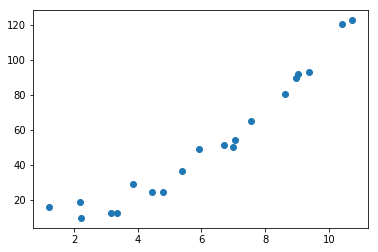In :
plt.scatter(x, y)
plt.axis([0, 100, 0, 100]);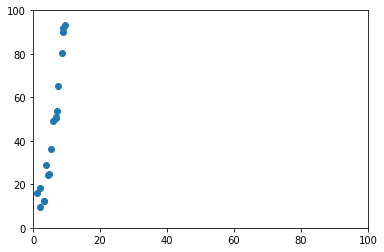Notice, the relationship in our data is $y \approx x^2$. To linearize our data, roughly speaking, we want to make $y$ "smaller" or make $x$ "bigger".

#### First, we'll look at the resulting plot when plotting $x$ vs $\log(y)$:¶

In :
plt.scatter(x, np.log(y))
plt.axis([0, 10, 0, 10]);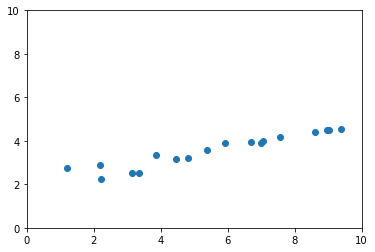This transformation did a decent job of bringing the magnitudes of $x$ and $y$ closer to one another. However, it's not perfect – it looks like the $x$ axis is significantly larger than the $y$ axis now. This is because the underlying relationship wasn't exponential (i.e. wasn't of the form $y \approx e^x$):

$$\log(y) = \log(x^2) = 2\log(x)$$

Our transformed plot effectively plots $x$ vs $2\log(x)$, which isn't linear.

#### Now, let's look at plotting $x^2$ vs $y$:¶

In :
plt.scatter(x**2, y);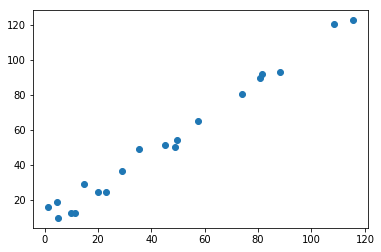This relationship is almost perfectly linear. This makes sense; our original plot was of $x$ vs $x^2$, and our new plot is of $x^2$ vs $x^2$.

#### And $x$ vs $\sqrt{y}$:¶

In :
plt.scatter(x, np.sqrt(y));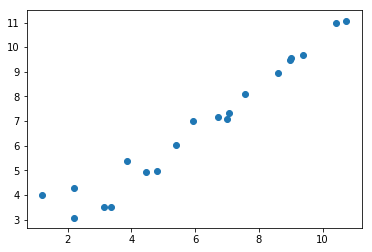This transformation accomplishes the same job as the previous. Instead of plotting $x$ vs $x^2$, we plotted $x$ vs $\sqrt{x^2}$, which (since we're only looking at non-negative $x$) is equivalent to plotting $x$ vs $x$. Note: Even though our plot has almost the exact same shape as the one in the previous plot, the axes are very different. Why is this the case?

#### Now, let's consider the plots of $\log(x)$ vs $y$ and $x$ vs $y^2$:¶

In :
plt.scatter(np.log(x), y)
plt.axis([0, 100, 0, 100]);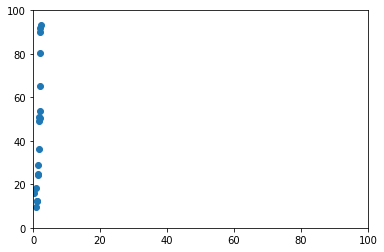In :
plt.scatter(x, y**2);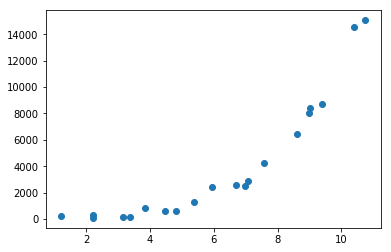The last two transformations had the opposite effect.

With $\log(x)$ vs $y$, the relationship we actually plotted was $y \approx (\log(x))^2$. In the latter, the relationship we plotted was $y \approx (x^2)^2 = x^4$ (note the scaled axes). Both of these transformations made the gap between the size of our inputs and size of our outputs greater, and neither of them resulted in a roughly linear plot.